# Controls - Stepper Motor, Load Cell and Solenoid Valves

Used in a coffee roastery as part of a larger system, but can easily be adapted for control needs. A lot of control in a small form factor.

IntermediateShowcase (no instructions)3 days1,059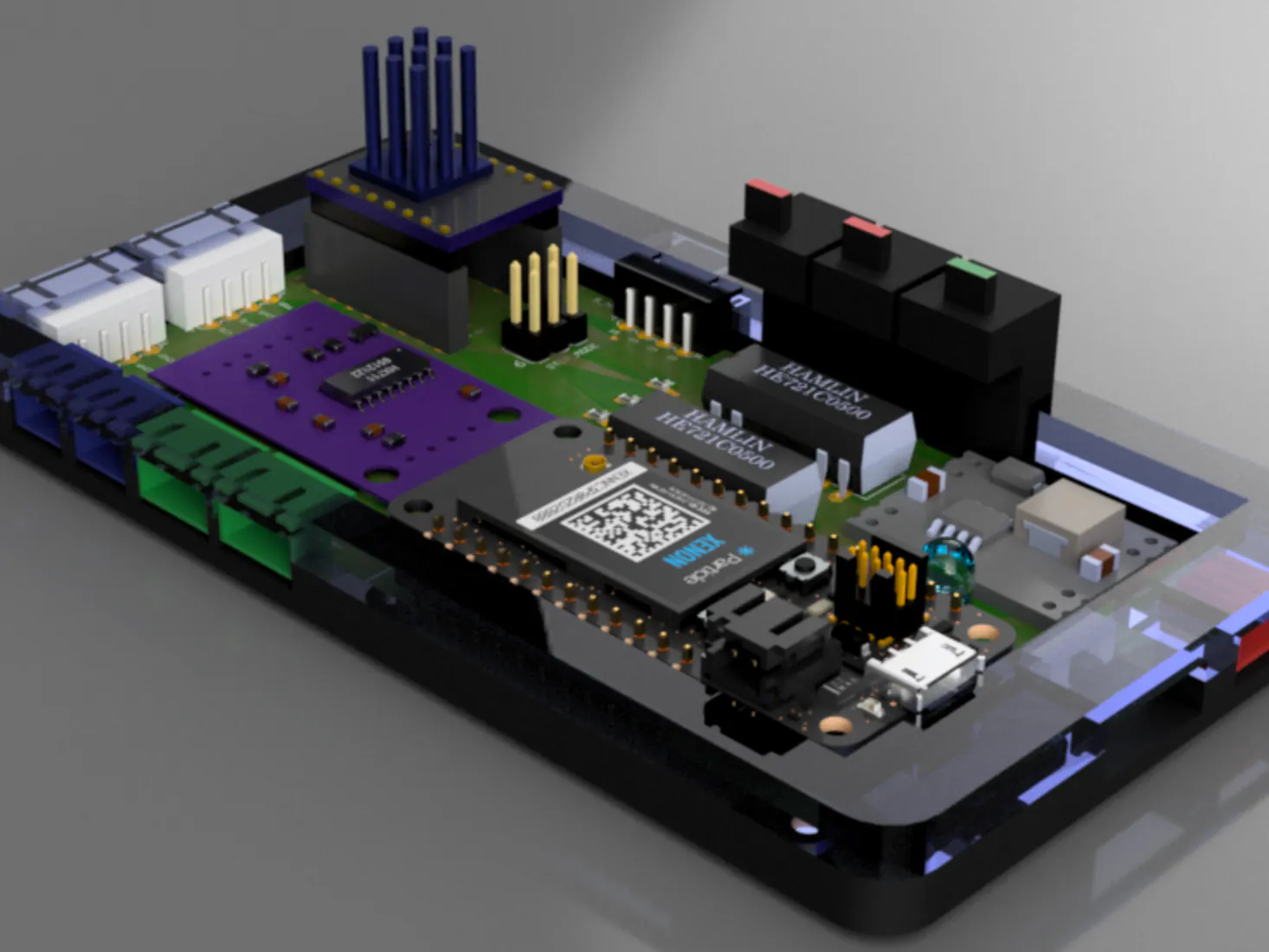## Things used in this project

### Hardware componentsPCBWay Custom PCB
×1
 Custom Plex-Glass enclosure
×1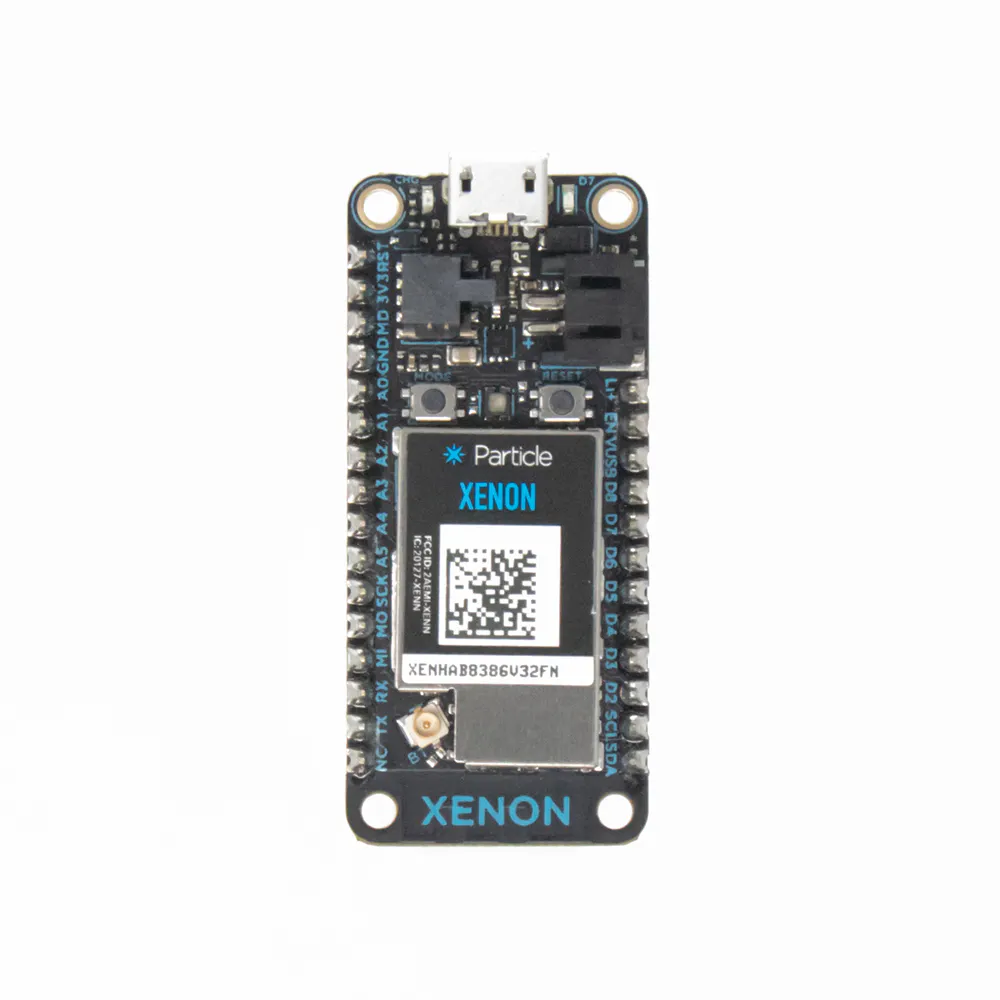Particle Xenon
×1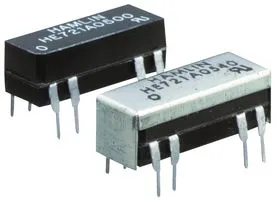Reed Relay, DPST-NO
×2
 TMC 2208 Silent Stepstick
×1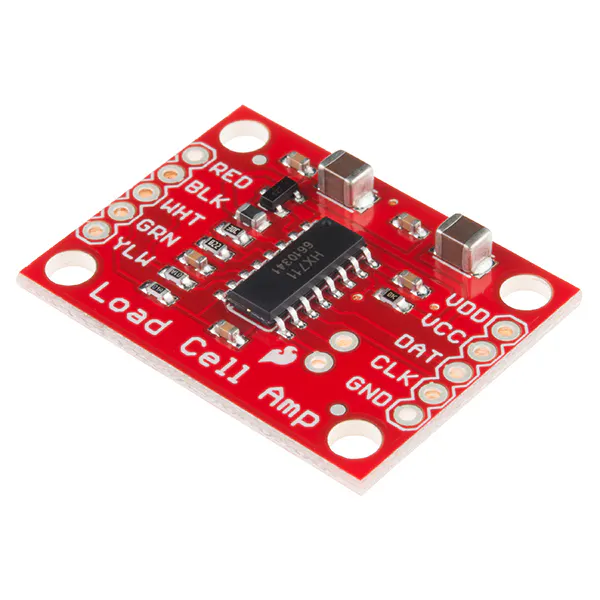SparkFun Load Cell Amplifier - HX711
×1
 Mini Stepown buck converter
×1
 5mm RGB led
×1
 Smalls - JST, SMD Resistors and LED
×1
 NEMA23 Stepper Motor
×1

### Software apps and online servicesBlynkAutodesk Fusion 360Autodesk EAGLEMicrosoft Visual Studio 2017Particle Build Web IDE

### Hand tools and fabrication machines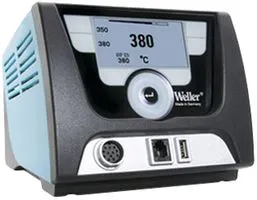Soldering Station Power Supply, For Weller WX Soldering System
 Panavise 350

Fusion 360

For XENON

For Xenon

## Code

### xenon-blynk-test.ino

C/C++
Code to be uploaded to Xenon
```// This #include statement was automatically added by the Particle IDE.
#include <spark-dallas-temperature.h>
#include <OneWire.h>
#include <Particle.h>

#define SCK D6
#define DT D7

#define dirPin A0
#define stepPin D0
#define ONE_WIRE_BUS A4                //  Temperature

#define stepsPerRevolution 3200

#define INTERVAL_MS 2000                // temp reasding interval

system_tick_t time_millis;              //

OneWire oneWire(ONE_WIRE_BUS);          //  Temperature
DallasTemperature sensors(&oneWire);    //  Temperature

int relay1 = D4;
int relay2 = D5;

int sensor2 = A5;                        // open to use

float Celsius = 0;                       // Temperature

double prevScaleValue;                  //INT SCALE CODE START
double scaleValue;
double fullWeight = 100;
double lockWeight;
bool locked = false;
int lockedInt = 0;

bool updatePrev = true;
double seenWeight;
int numTimesSeen;

int tareScale(String command);
double absValue(double value);
int setFullWeight(String command);
int lockScale(String command);          //INT SCALE CODE END

void setup() {

Serial.begin(9600);                                  //SETUP SCALE CODE START
Particle.variable("scaleValue", scaleValue);
Particle.variable("lockedInt", lockedInt);
Particle.function("setFull", setFullWeight);
Particle.function("tareScale", tareScale);
Particle.function("lockScale", lockScale);

scale.set_scale(107.32);
Serial.println(scale.get_units());
scale.tare();                                     //SETUP SCALE CODE START

time_millis = 0;                                     // Set time to 0

pinMode(A4, INPUT);
pinMode(A5, INPUT);

RGB.mirrorTo(D3, D2, D1);

pinMode(stepPin, OUTPUT);
pinMode(dirPin, OUTPUT);

pinMode(relay1, OUTPUT);
pinMode(relay2, OUTPUT);

Particle.subscribe("Solenoid1", myHandler1);
Particle.subscribe("Solenoid2", myHandler2);
Particle.subscribe("Stepper", myHandler3);

sensors.begin();

}

void myHandler1(const char *event, const char *data) {

if (strcmp(data,"On")==0) {
RGB.control(true);
RGB.color(255, 0, 0);
digitalWrite(relay1, HIGH);

}else if (strcmp(data,"Off")==0) { {
RGB.control(false);
digitalWrite(relay1, LOW);
}
}
}

void myHandler2(const char *event, const char *data) {

if (strcmp(data,"On")==0) {
RGB.control(true);
RGB.color(0, 0, 255);
digitalWrite(relay2, HIGH);

}else if (strcmp(data,"Off")==0) { {
RGB.control(false);
digitalWrite(relay2, LOW);
}
}
}

void myHandler3(const char *event, const char *data) {

if (strcmp(data,"On")==0) {
StepperMotor();

}else if (strcmp(data,"Off")==0) { {

digitalWrite(stepPin, LOW);
RGB.control(false);

}
}
}

void loop() {

temperature();
LS();                                    //LOOP SCALE CODE
}

void StepperMotor()

{
RGB.control(true);
RGB.color(255, 255, 255);

for (int i = 0; i < 5 * stepsPerRevolution; i++) {
digitalWrite(dirPin, HIGH);

digitalWrite(stepPin, HIGH);
delayMicroseconds(100);
digitalWrite(stepPin, LOW);
delayMicroseconds(100);
}
}

void temperature() {

sensors.requestTemperatures();
Celsius = sensors.getTempCByIndex(0);

if( millis() - time_millis > INTERVAL_MS ) {               //Set time to the 'current time' in millis
time_millis = millis();

int rand = random(20,30);                               // Create a random number
Mesh.publish("Temp",String::format("%d",rand));     // Publish our "temperature" value

}

}

void  LS() {                          //FUNCTION SCALE CODE START
scale.power_up();

if(scaleValue > prevScaleValue+2 || scaleValue < prevScaleValue - 2){
Mesh.publish("weightChange");
}

if(locked && (scaleValue > prevScaleValue +2 || scaleValue < prevScaleValue -2)){
updatePrev = false;
numTimesSeen++;
if(numTimesSeen >=2){
updatePrev = true;
numTimesSeen = 0;
Mesh.publish("lockWtChange");
}
}

if(updatePrev){
prevScaleValue = scaleValue;
}
scaleValue = scale.get_units(5);
scaleValue = absValue(scaleValue);

scale.power_down();
int startTime = millis();
while(true){
if(millis()-startTime >= 500){
break;
}
}

}

int tareScale(String command){
scale.tare();
delay(100);
Serial.println("Scale Teared From Cloud!!");
return 1;
}

int setFullWeight(String command){
fullWeight = scaleValue;
return fullWeight;
}

int lockScale(String command){
locked = !locked;
lockWeight = scaleValue;

if(locked){
lockedInt = 1;
return lockWeight;
}else{
lockedInt = 0;
return -1;
}
}

//Not really working right now
double absValue(double value){
double newValue = 0;
if(value < 0){
newValue = -1*value;
return newValue;
}
return value;

}                                       //FUNCTION SCALE CODE END
```

### Blynk_Argon.ino

C/C++
Argon Code
```#include <blynk.h>

char auth[] = "Your_Blynk_Code"; // Puppy

int Solenoid1;
int Solenoid2;
int Stepperrun;

int debug = D7;

void setup() {

Serial.begin();
Blynk.begin(auth);

Mesh.subscribe("Temp",tempHandler);      //sensor one data received from Xenon - in use
Mesh.subscribe("Temp2",tempHandler2);    //sensor two data received from Xenon - available

Mesh.subscribe("weightChange",W1Handler);   //LS one data received from Xenon - in use
Mesh.subscribe("lockWtChange",W2Handler);   //LS two data received from Xenon - in use

pinMode(debug, OUTPUT);
}

BLYNK_WRITE(V1)
{
Solenoid1 = param.asInt();            // Assigning incoming value from pin V1 to variable
if (Solenoid1 == 1) {
Particle.publish("Solenoid1", "On");
digitalWrite(debug, HIGH);

}else if (Solenoid1 == 0) {
Particle.publish("Solenoid1", "Off");
digitalWrite(debug, LOW);
}

delay(100);

}

BLYNK_WRITE(V2)
{
Solenoid2 = param.asInt();            // Assigning incoming value from pin V2 to variable

if (Solenoid2 == 1) {
Particle.publish("Solenoid2", "On");

}else if (Solenoid2 == 0) {
Particle.publish("Solenoid2", "Off");
}
delay(100);

}

BLYNK_WRITE(V3)
{
Stepperrun = param.asInt();

if (Stepperrun == 1 ) {                    // Do Stepper Motor stuff
Particle.publish("Stepper", "On");

} else {
Particle.publish("Stepper", "Off");
}

delay(100);

}

void loop() {
Blynk.run();
}

// Sensor one data sent to Blynk//
void tempHandler(const char *event, const char *data){
Serial.printlnf("event=%s data=%s", event, data ? data : "NULL");
Blynk.virtualWrite(V0, data);
}

// Sensor two data sent to Blynk//
void tempHandler2(const char *event, const char *data){
Serial.printlnf("event=%s data=%s", event, data ? data : "NULL");
Blynk.virtualWrite(V5, data);
}

// LS one data sent to Blynk//
void W1Handler(const char *event, const char *data){
Serial.printlnf("event=%s data=%s", event, data ? data : "NULL");
Blynk.virtualWrite(V6, data);
}
// LS two data sent to Blynk//
void W2Handler(const char *event, const char *data){
Serial.printlnf("event=%s data=%s", event, data ? data : "NULL");
Blynk.virtualWrite(V7, data);
}
```

## Credits

### FireFli (PTY) LTD

10 projects • 12 followers
New to this... but loving it :)
Thanks to Louis Pieterse and Ben Dixon.